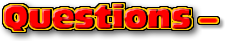ChaptersThis text is meant to accompany class discussions. It is not everything there is to know about the basics of electricity. It is meant as a  prep for class. More detailed notes and examples are given in the class notes, presentations, and demonstrations (click here.)Click for the questions that go with this reading
Power

When studying mechanical energy, power was defined as how quickly energy is delivered or received. The cool thing about energy is that it and its related definitions are always the same. This means that power is still define as how quickly energy is delivered or received.Voltage is define asCurrent is defined asCurrent times the voltage isthereforeP is power measured in Watts [ W ]
I is the current measured in Amps [ A ]
V is the voltage measured in Volts [ V ]

 Example A 2.5 Watt night light is plugged into a wall outlet. What is the resistance of the light bulb?

Other equations for power can be created by combining Ohm's Law with the power formula.Thermal Power/Energy LosesIn a circuit made of a battery and a MP3 player. The battery powers the MP3 player. Charges carry energy to the MP3 player. When they return to the other side of the battery with less energy it is obvious where much of the power went. It went in to making music play through the headphones and reading the music from a computer chip and powering the buttons and lights you pressed to select the song. And a little bit went into heating up the device. This last energy is a thermal energy loss.

Ohmic devices lose nearly all of their energy in form of thermal energy. When the power is calculated for any of these devices it is radiated as thermal energy. It is wasted energy -unless you are cooking or heating up a space.

The electric company is well aware of the power losses when transmitting power along high tension power lines. Suppose the electric company wants to deliver 500,000 Watts of power to your community. On one end of the line they put 500,000 Watts of power into the wires. They need make sure the most of that power gets to the other end and is not lost in heating up the wires.

The wires have a resistance in them that is constant. The electric company can control the amount of voltage and current they put into the lines. By putting in the energy as a high voltage, like 500,000 Volts the current would 1 Amp. The dissipated in wires is calculated according to the equation below. (Any equation would work for calculating power, but this one has only current and resistance in it.)Since the current is squared and multiplied by the wire's resistance, if the current is reduced then the thermal energy losses in the power lines are minimalized. If 1 volt was put through the power line, then to get the same 500,000 W of power the current world have to be 500,000 A. This large amount of a current would probably cause the wires to glow! ...and they would have to be considerably shorter than they are.

 Example A power line with a wire diameter of 2.2 cm carries a current of 0.1 amps across a distance of 1.00 km. If the wire is made from aluminum, then how much power is lost as thermal energy?

by Tony Wayne ...(If you are a teacher, please feel free to use these resources in your teaching.)